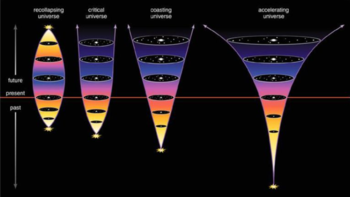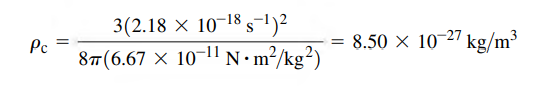Critical density of Our UniverseFrom Hubble-Lemaitre law1, we got to know that our universe is expanding and gives the relation between recessional velocity of galaxies and distance from us as,where H0 is Hubble constant , v is recessional velocity and r is distance between galaxy and us.

In the universe, Gravitational force is the major force that exists at this large scale. From Newtonian Mechanics, we know that the gravitational force is only attractive force  but it can also be repulsive. This phenomenon is described by the inflationary theory which is not proved yet. It's natural to think that  in an expanding universe, gravitational attraction between galaxies could somewhat slow initial expansion. If these attractions are strong enough that  it can slow down the expansion slowly and slowly that in future it can stop the expansion and begin to contract which leads to another big bang, this phenomenon is known as Big Crunch.  Another phenomenon would be ,'The universe is expanding indefinitely forever.'

The above mentioned phenomena will occur or not depends on the average density of matter. If matter is relatively dense, then the gravitational attraction is enough to slow and stop the expansion of the universe and make the universe contract again. If that happens, then we can calculate the critical density up to which the universe can reach from Newtonian Mechanics. Since, it is based upon the Newtonian Mechanics, it isn't relativistically correct. The process to find the critical density of the universe is quite analogous to finding escape speed of a projectile launched from the earth.

Before that let's have a insight about the cosmological principle which tells that the Universe looks same from all positions in space apart from some local irregularities which means that in a large  enough volume, the average density is constant.A large sphere with radius R and total mass M, containing many galaxies is considered. Suppose our milky way galaxy with mass m, is on the surface of the sphere. The resultant gravitational force that is exerted by the uniform distribution of mass out of the sphere is zero which is a consequence of the cosmological principle.

We all know, the density of matter is just the mass divided by its volume. Then the total mass inside the sphere can be calculated asNow, the total energy of our galaxy is,If E<0, then we are heading towards the center of the sphere which means universe is contracting where as if E>0 , then we  expelled from the sphere meaning that the universe is expanding indefinitely. But at the condition of critical density of the universe, there is neither expansion nor contraction, so, E=0. Then,Substituting the value of v from Hubble's law and M from  definition of density we getRearranging the term we get,The above relation is for critical density of our Universe.

Measuring the accurate value of Hubble constant is most challenging problem in Cosmology till now which is often considered as Crisis in Cosmology. Nevertheless, the current best value of this constant is 2.18 x 10-18s-1.

So, putting the value of H0 and G we get value for critical density for our universe,The mass of a hydrogen atom is 1.67 x 10-27kg, so this density is equivalent to about five hydrogen atoms per cubic meter, that's quite unbelievable , isn't it?

1. In 1927 AD, before two years of Edwin Hubble's work, an astronomer named 'Georges Lemaitre', was the first to publish research deriving the law.

You can check these videos from physicists too: